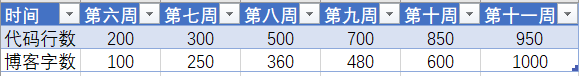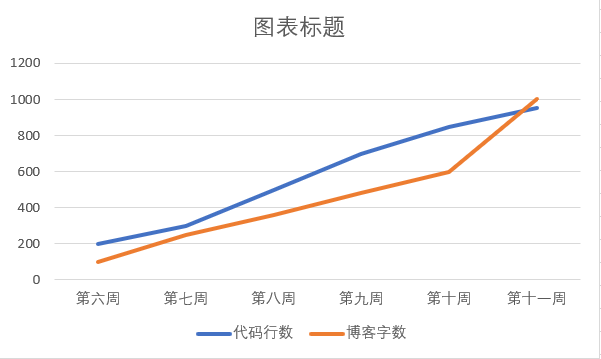# 第六次作业

## 7-1 求1到100的和

#include<stdio.h>
int main()
{
int i=1,sum=0;

for(i=1;i<=100;i++){
sum=sum+i;
}
printf("sum = %d",sum);
return 0;
}


思路：

1）定义两个变量，一个循环变量，一个求和变量；

2）让求和变量的初始值为零，让循环变量从一递增到一百并逐一求和；

3）设置一个判断值使得循环变量不会提前退出循环。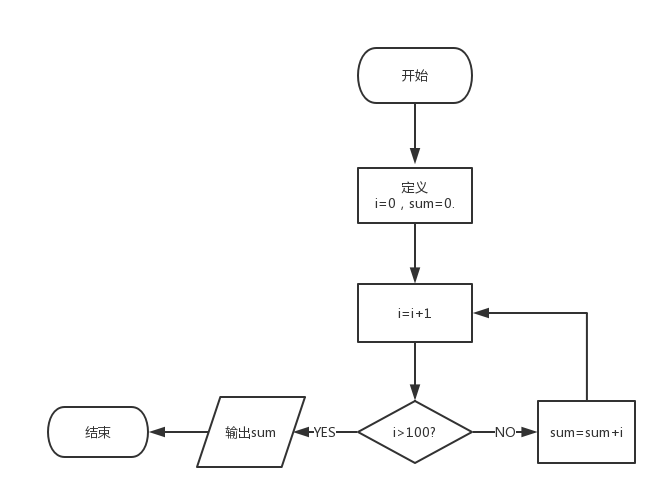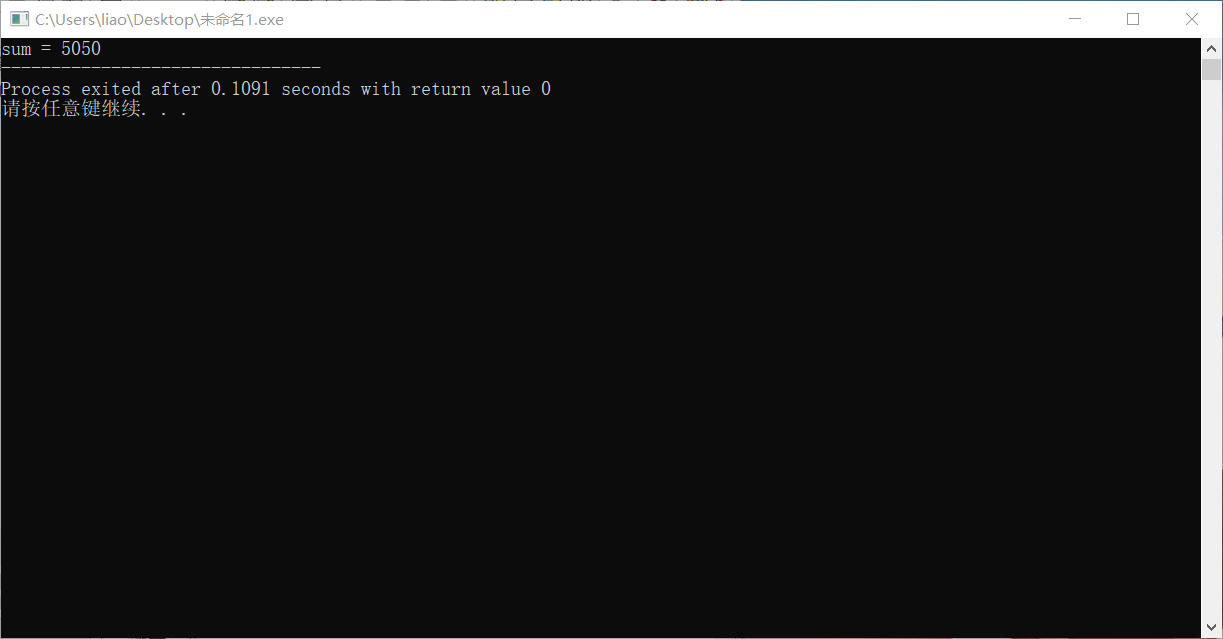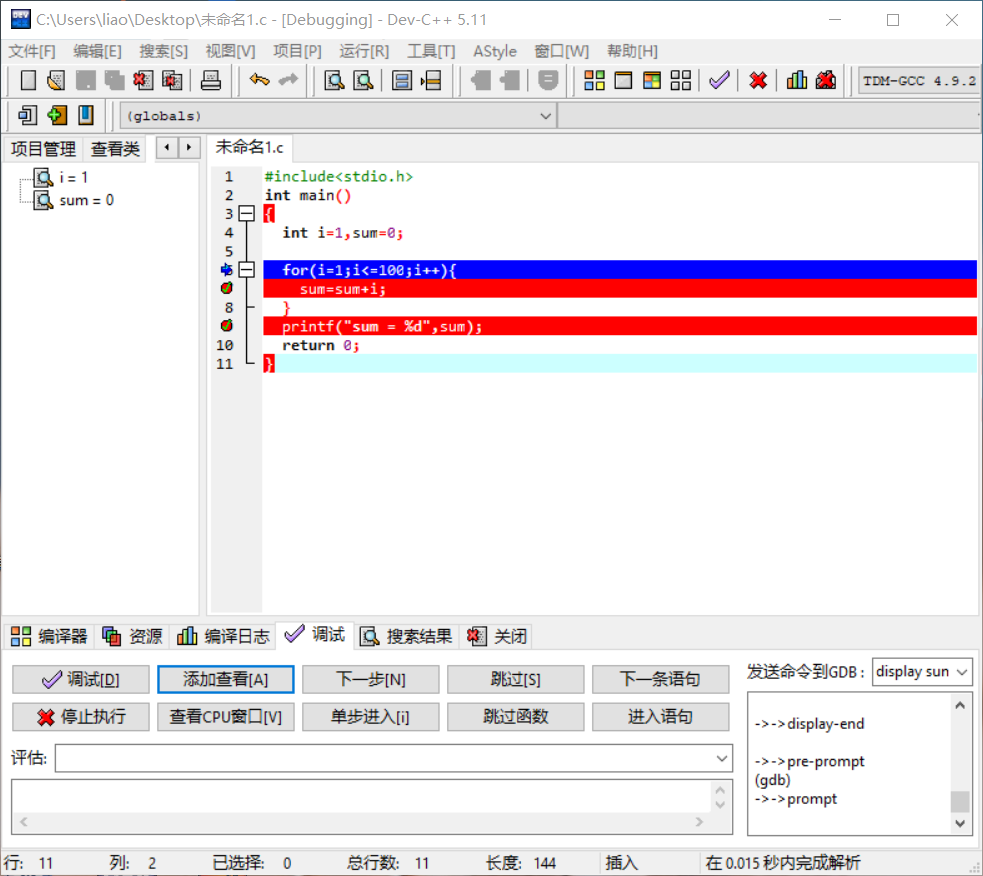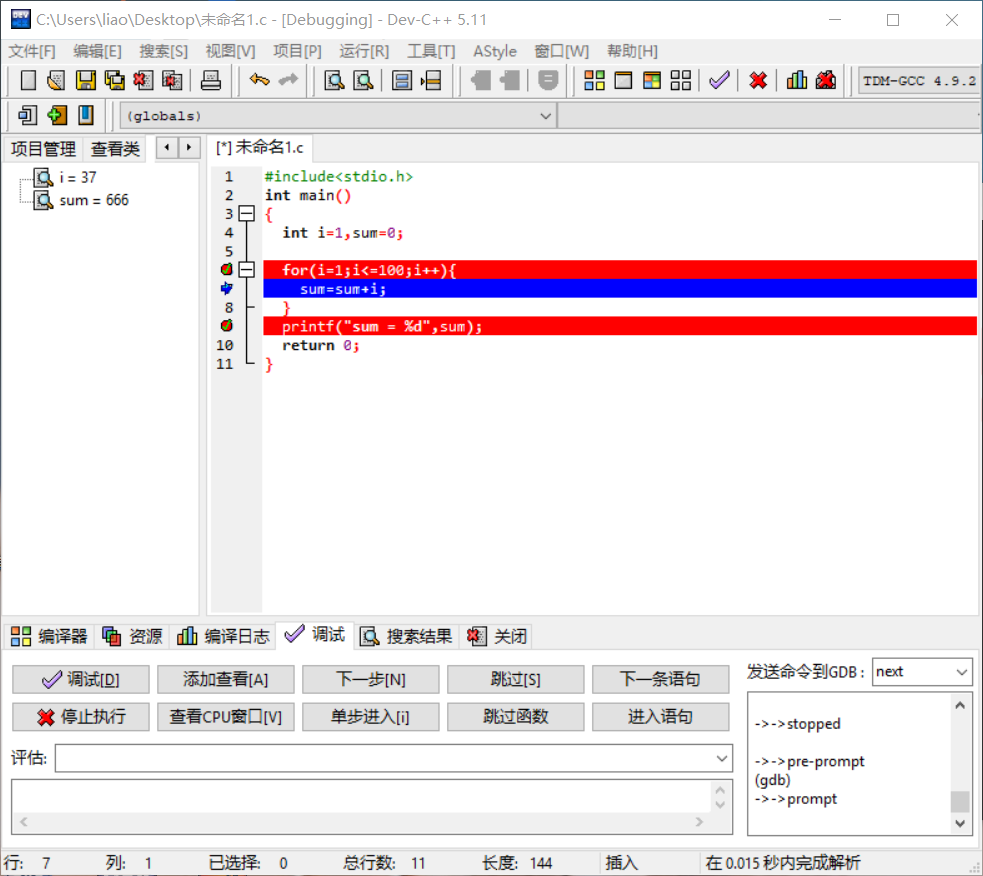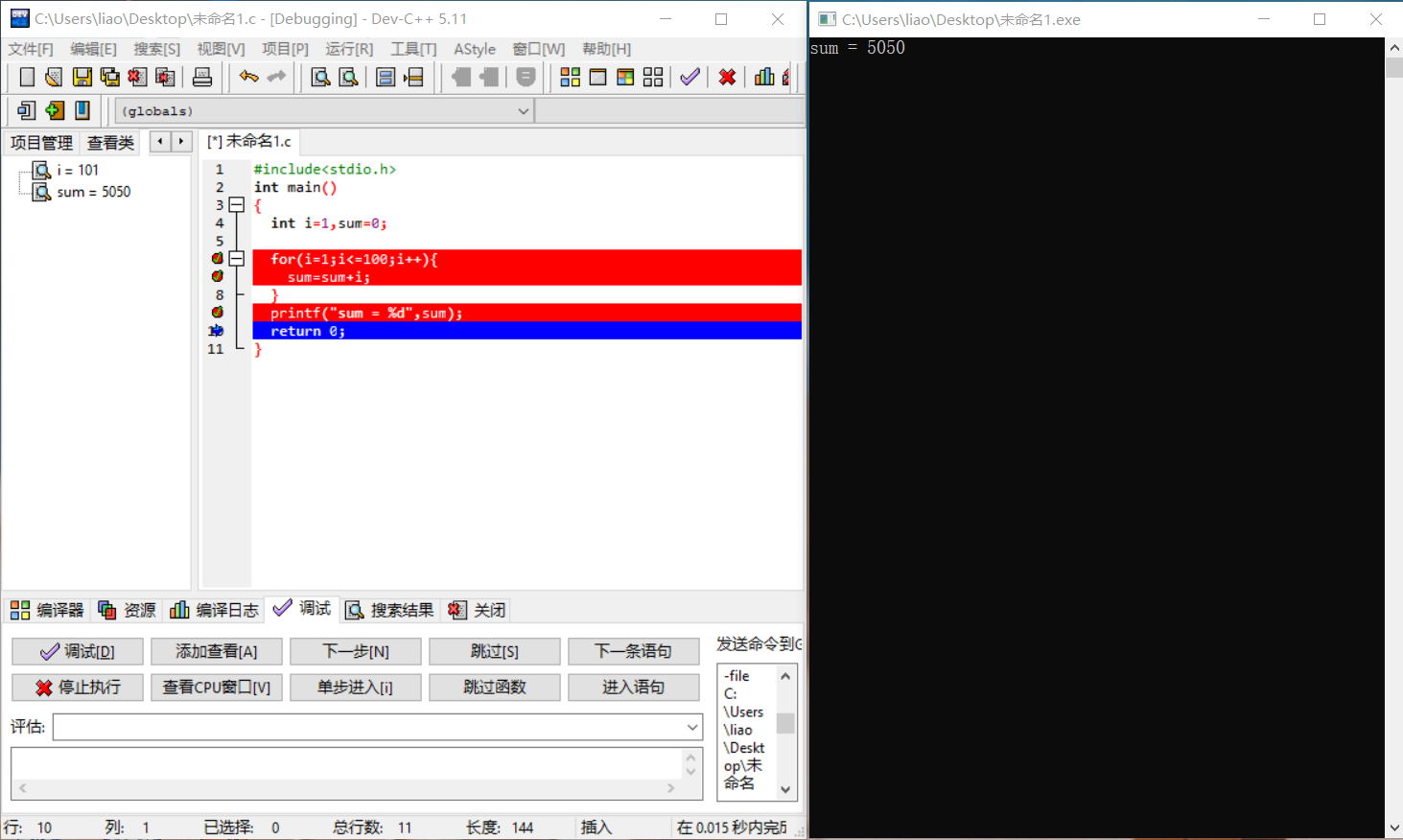# 第九次作业

## 7-1 求整数的位数及各位数字之和

#include <stdio.h>
int main()
{
int b=0,n,sum=0;
scanf("%d",&n);
do
{
sum+=(n%10);
b++;
n/=10;
}
while(n!=0);

printf("%d %d",b,sum);
return 0;
}


1）首先定义一个输入数，一个求和数，一个计数变量；

2）将输入的变量进行运算，使输入的n每次循环时都除以10，并让sum每次都加上n，最后让计数变量每次加一，完成每次运算；

3）循环时当n为零时结束循环。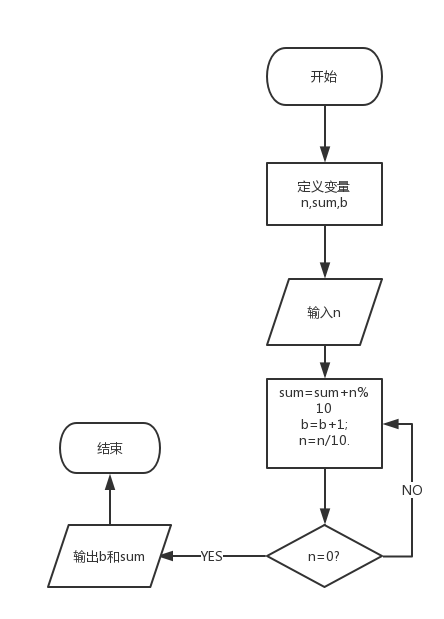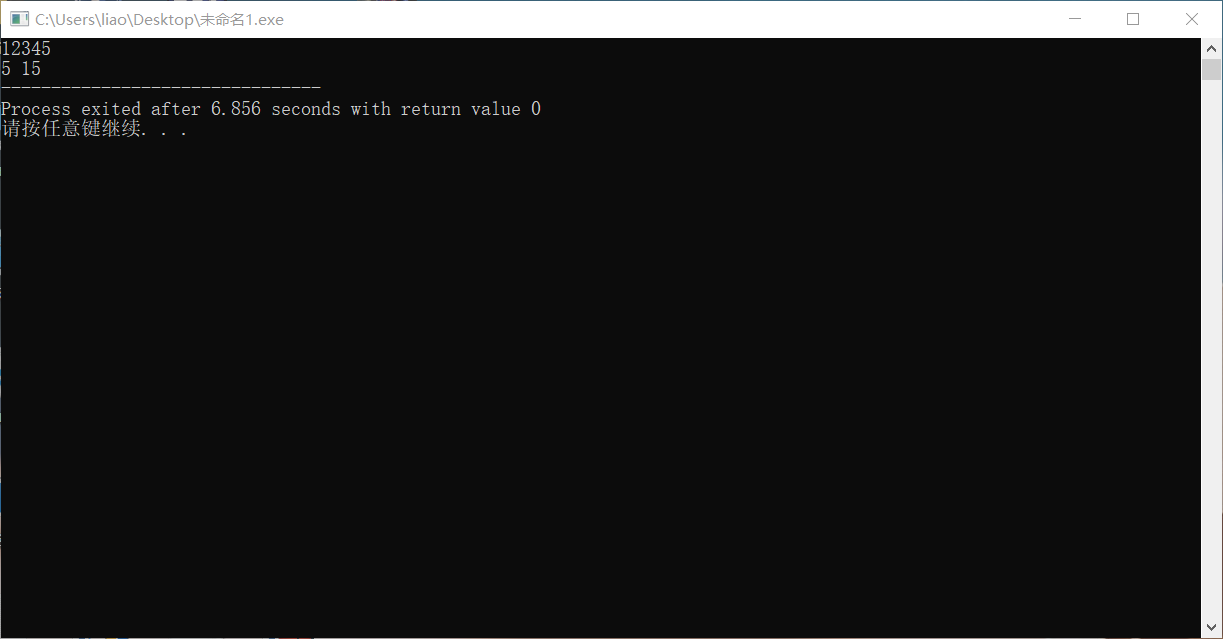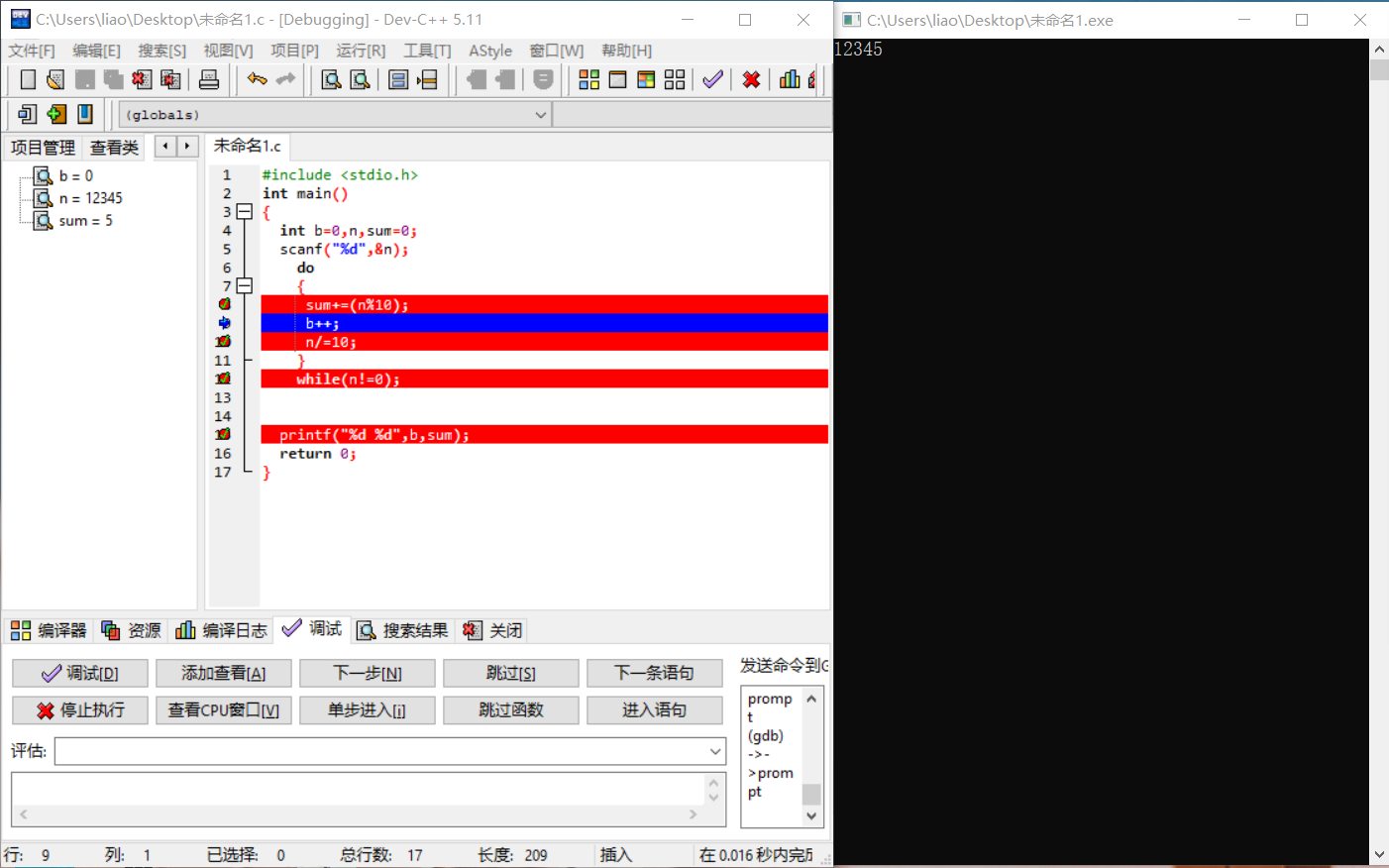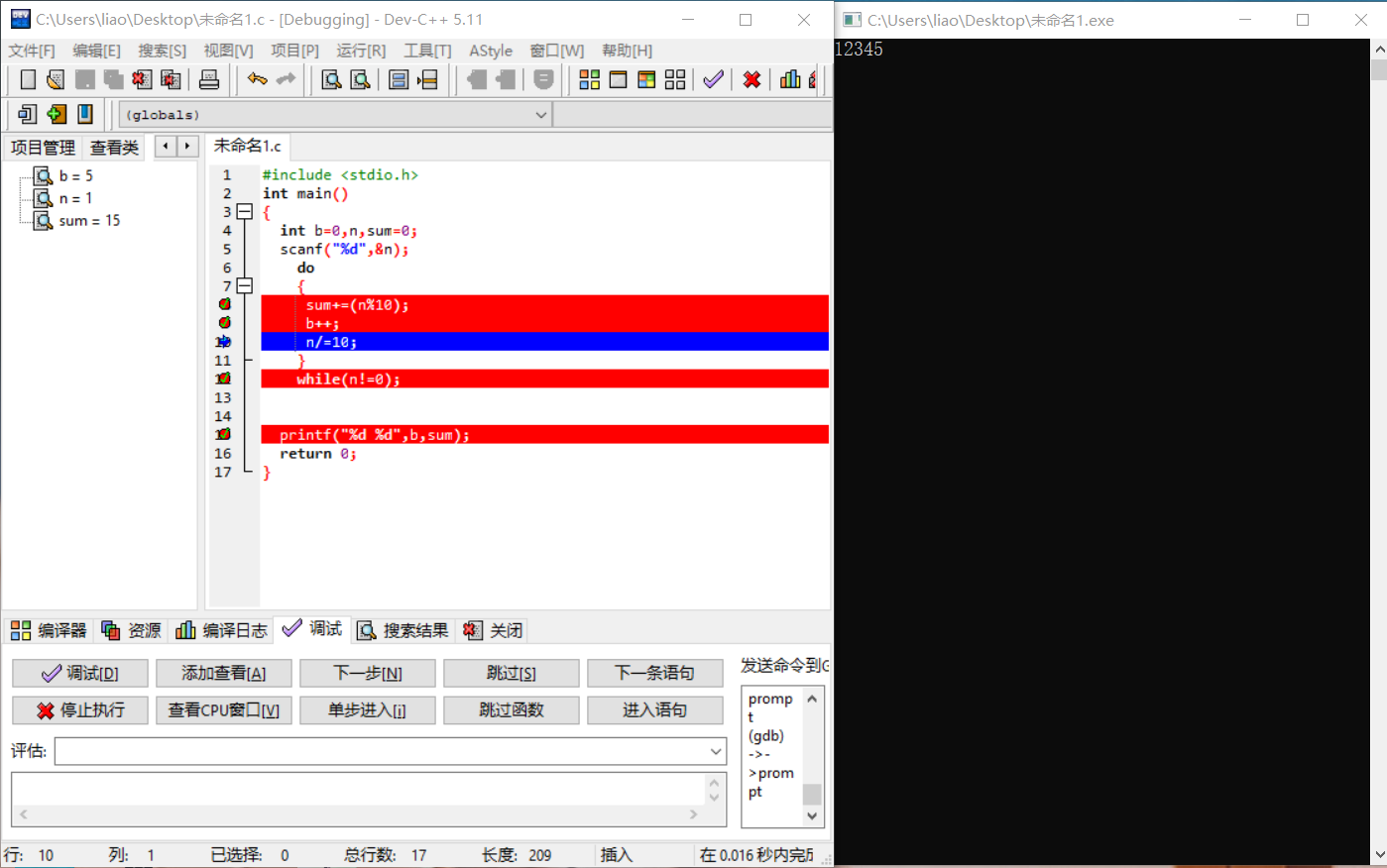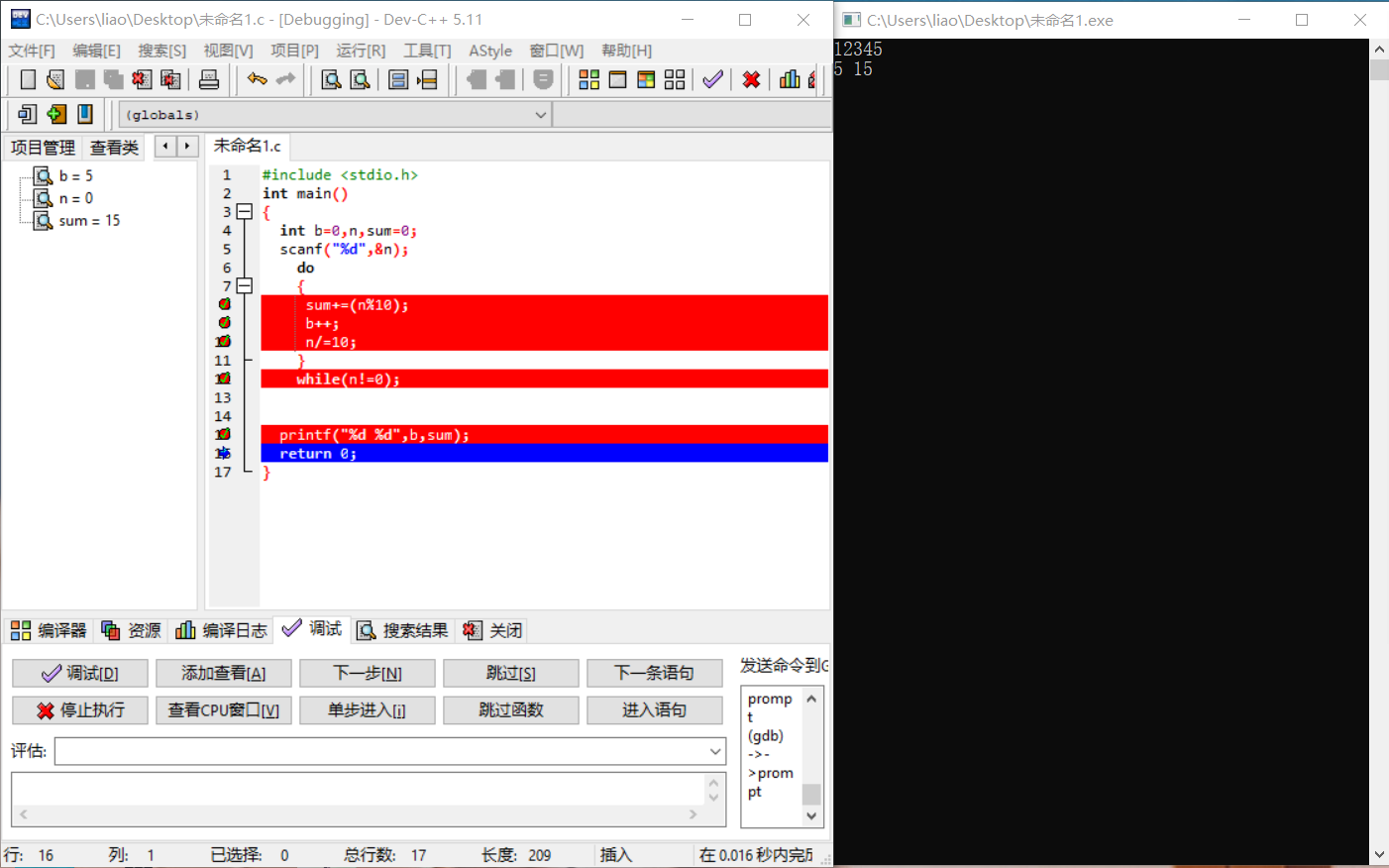# 第十次作业

## 7-1 统计素数并求和

#include<stdio.h>
int main()
{
int M,N,i,j,count=0,sum=0,flag=0;
scanf("%d %d",&M,&N);
for(i=M;i<=N;i++){
for(j=2;j<i;j++)
{
if(i%j==0)
{
flag=1;
break;
}
}
if(flag==0)
{
count++;
sum=sum+i;
}
flag=0;
}
if(M==1)
{
printf("%d %d",count-1,sum-1);
}
else
{
printf("%d %d",count,sum);
}
return 0;

}


解题思路：

1）首先定义两个输入量最小值M和最大值N，再定义一个计数变量count和一个两个控制循环变量i和j，接下来定义一个求和变量sum，最后定义一个标志变量flag；

2）输入最小值最大值确定范围；

3）让i从M开始循环，让j从2开始循环；

4）让i除以j取余，如果余数为零则证明i对应的数为非素数，这时将初始值为0的flag赋值为1，使用break跳出j的循环；

5）在i的循环里进行判断，如果flag等于0，则使count加一且让sum等于原值加上这时的i；

6）在i的循环最后将flag的值还原为0；

7）如果M的值为1，因为1并不是素数但计算机又已经将1视为素数进行运算，所以将最后输出的两个值分别减1。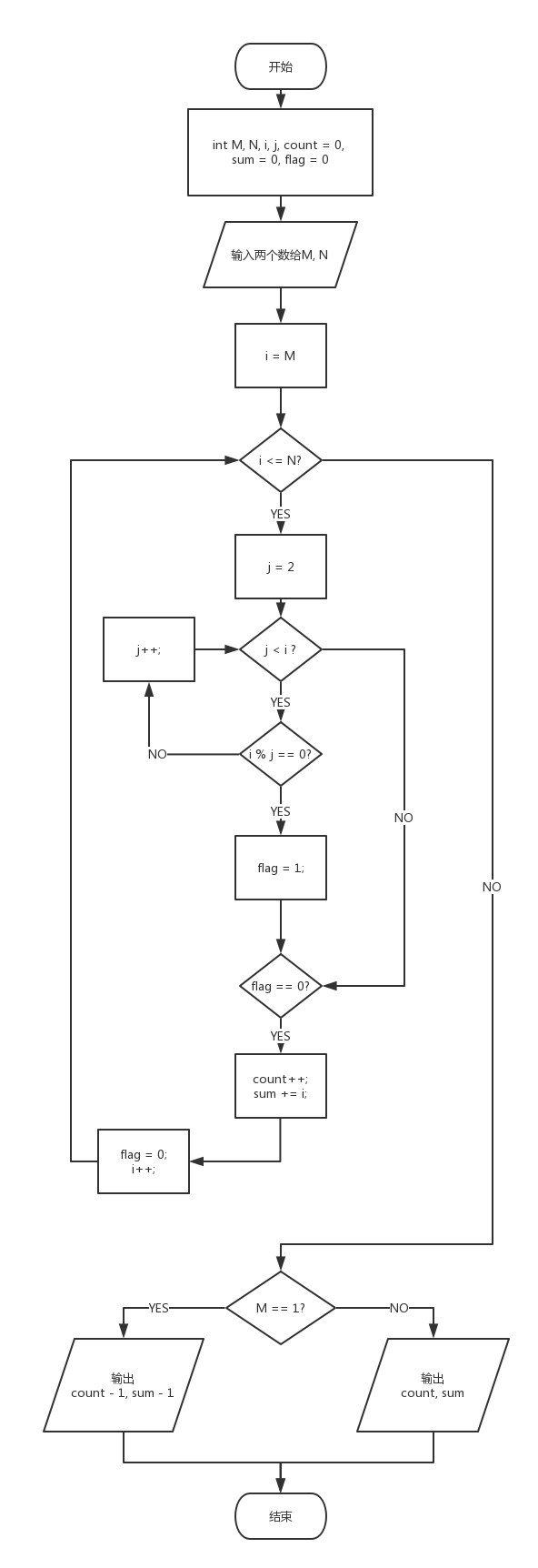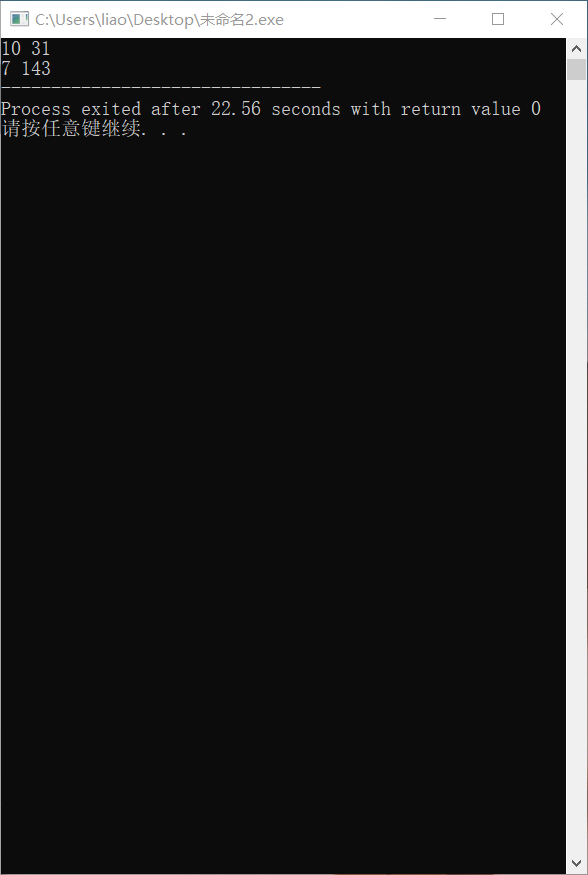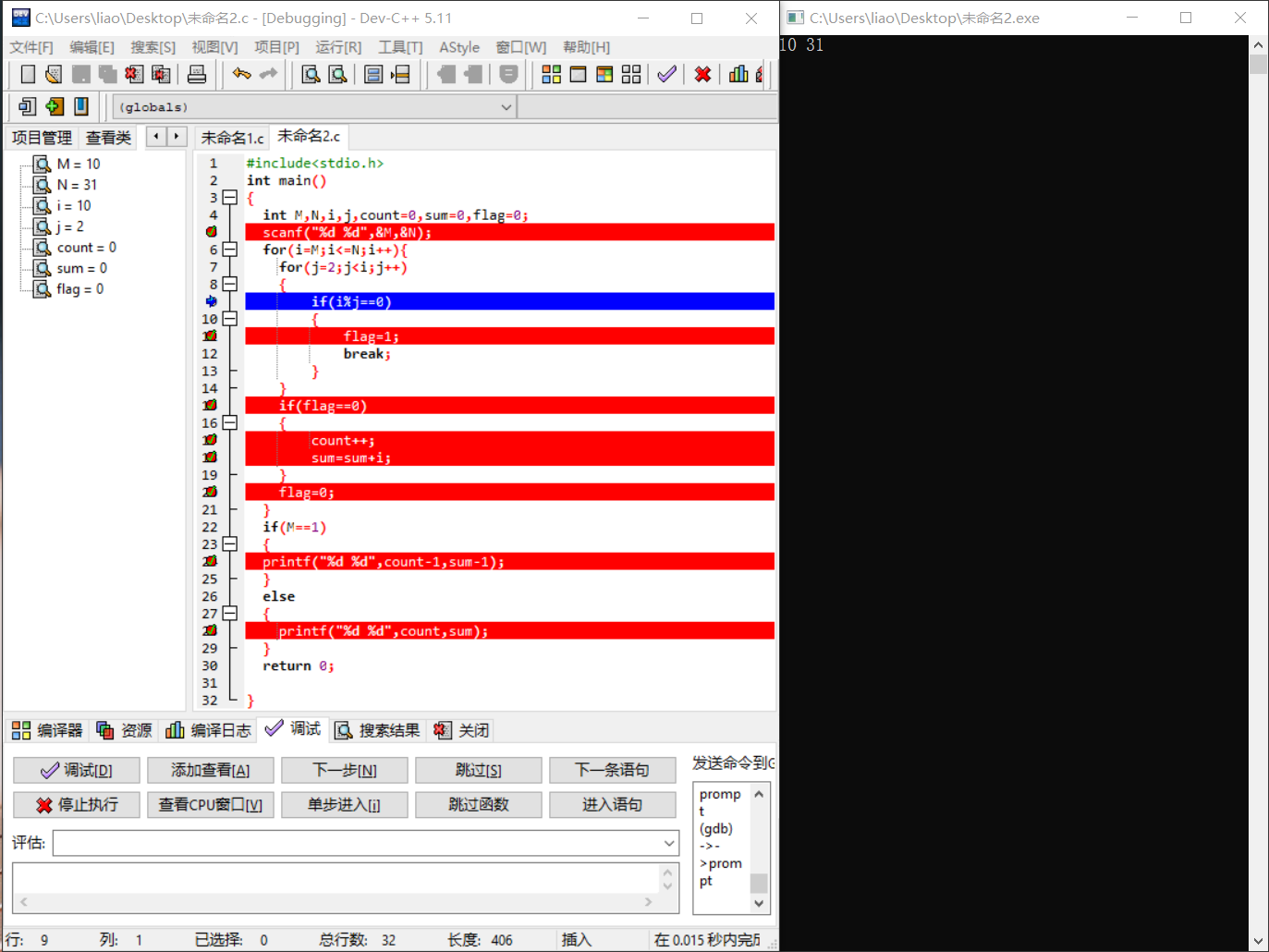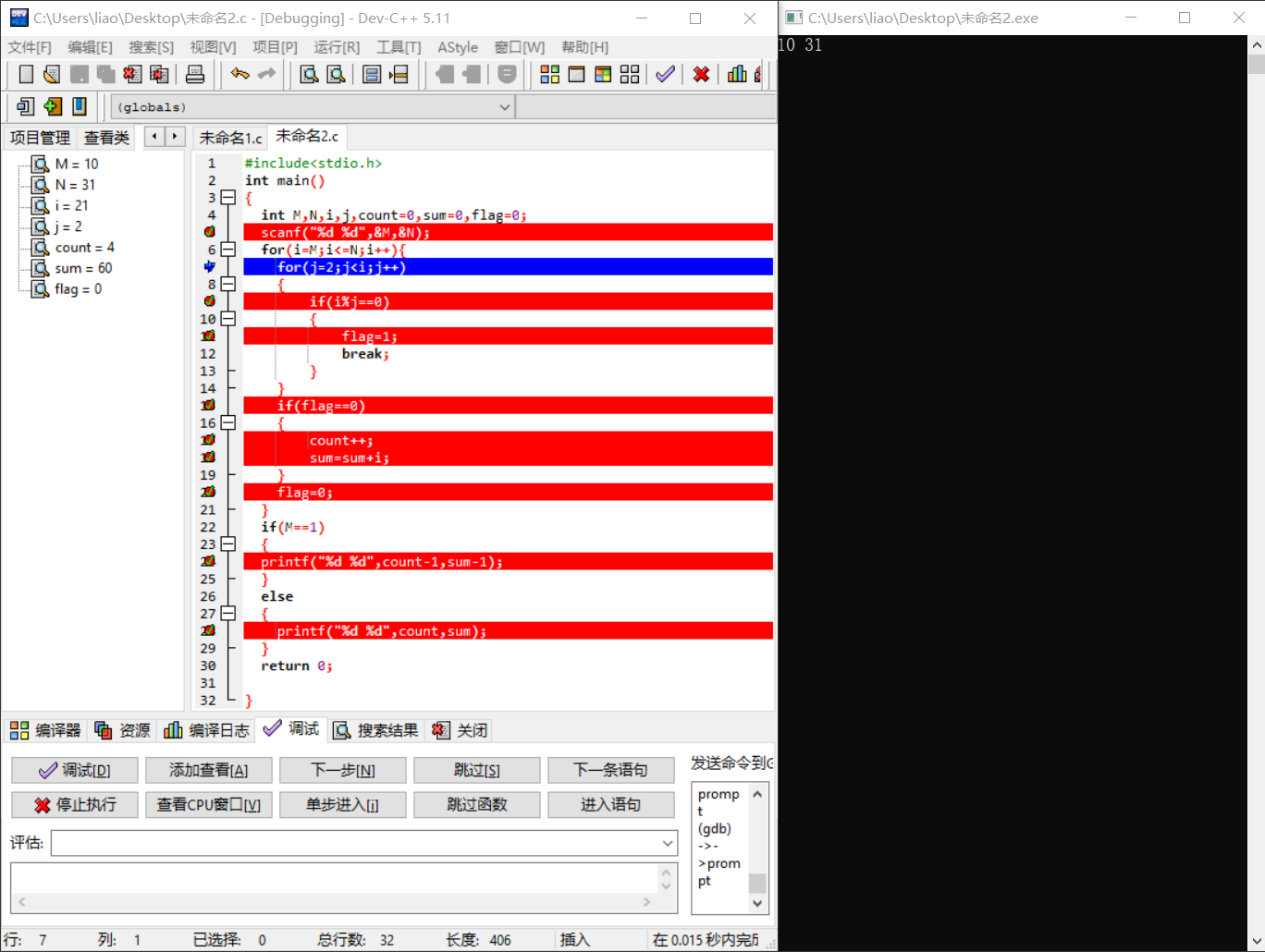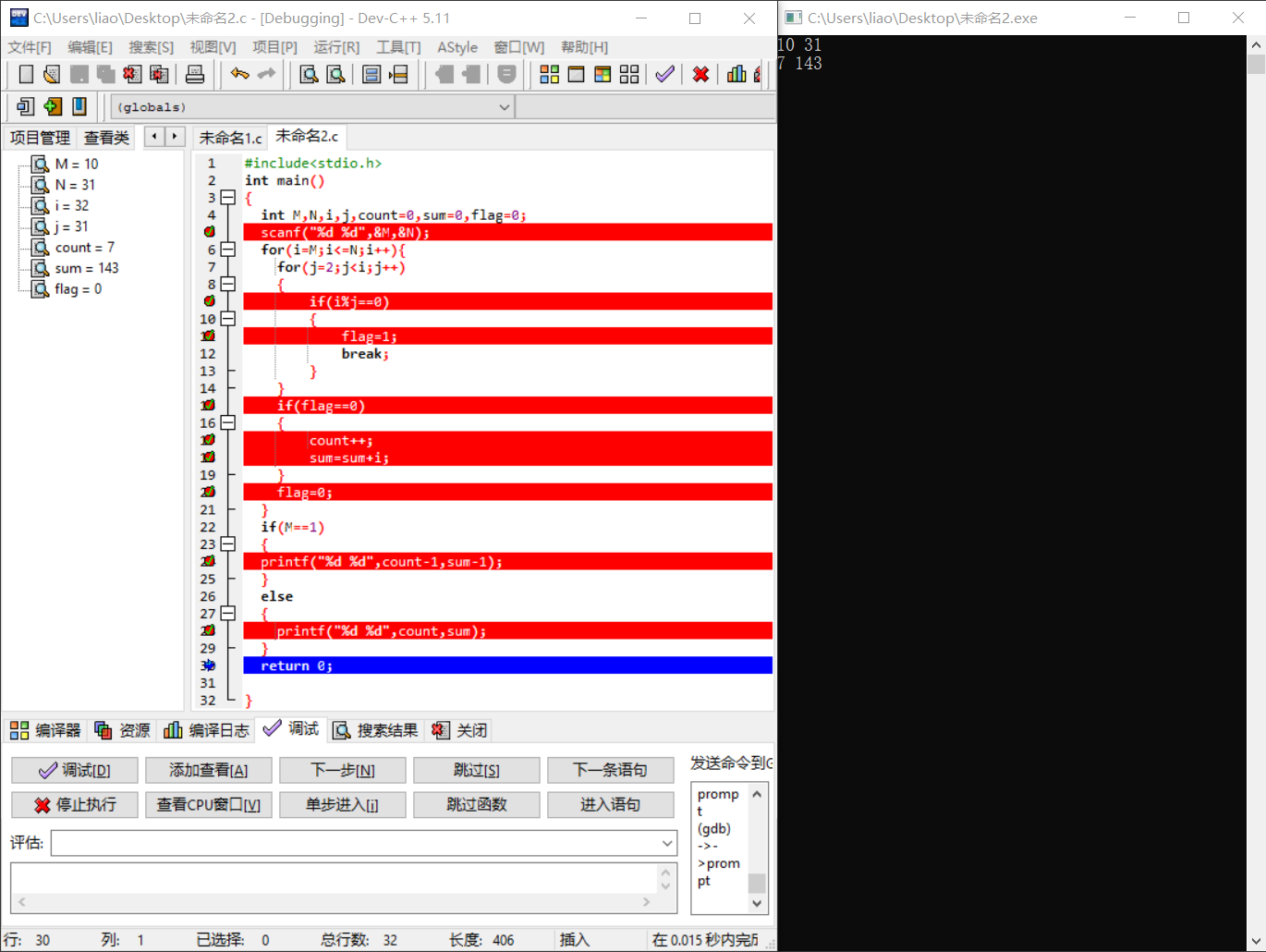### 学习进度条